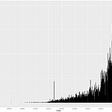# R Language for The Project Management Course — AUG University “part 2”

Welcome back! This is the 2nd chapter of the series explaining the project management course for AUG students - The computer system engineering department.

# Descriptive Statistics:

## First, what is descriptive statistics?

Descriptive statistics summarize or describe the characteristics of a data set. Data set is a collection of responses or observations of a sample or entire population.

## Types of descriptive statistics

There are 3 main types of descriptive statistics:

1. The distribution: concerns the frequency of each value.
2. The central tendency: concerns the averages of the values.
3. The variability: concerns how to spread out the values are.

## - Central tendency:

Measures of central tendency estimate the average of the data set. The mean, median, and mode are 3 ways of finding the average.

we will talk about mean and median:

## Mean:

we calculate the mean by dividing the sum of all values by the total number of values.

`data set : 11 ,22, 33, 44, 55, 66mean is : (11+22+33+44+55+66) /6`

In R, we can calculate the mean using the mean(x) function, x is a vector.

## Median:

we can calculate the median in R using median(x)

`data set : 11 ,22, 33, 44, 55, 66median is :  n = 6              since n is even > median is (x(n/2)+ x((n/2) + 1))/2                             > median is (x 3 + x 4) /2                              > median is (33 + 44) /2                             > median is  38.5`

another example:

`data set : 11 ,22, 33, 44, 55, 66, 77median is :  n = 7             since n is odd > median is (x(n+1))/2                             > median is x(7 +1) /2                             > median is x(4)                             > median is  44`

## Standard deviation:

is a measure of the amount of variation in a set of data. In R we use sd(x) to measure the standard deviation.

## Variance

Is the average of squared deviations from the mean. In R we measure it by using var(x)

We can calculate the standard deviation and variance using:

`data is 10, 20, 30step 1: Mean is (30 +20 +10)/3 = 20                -----------------------------------step2:Raw data              Deviation from mean      Squared deviation10                         10 - 20 = -10         (-10)^2 = 10020                         20 - 20 = 0               030                         30 - 20 = 10             100                            sum = 0                sum = 200Now to calucate the variance you have to calucate√(sum of sqared deviation / n-1) then the vairnce(result ^2)Step 3: sum of sqared deviation / n-1 = 200/2 = 100Step 5: √100= 10`

this means that each score deviates from the mean by 10 points.

`Now varince is 10^ 2 = 100`

See you in the next part;)

--

--

--

Hunger for Knowledge, adventure, and risk enthusiastic. Software engineering student

Love podcasts or audiobooks? Learn on the go with our new app.

## The funnel is one of my favorites.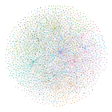## Here is the reason why SQLAlchemy is so popular.## Defining, Predicting, and Preventing Disengaged Users in FinTech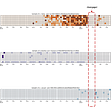## Wine Reviews Dataset: Exploration (Part 1)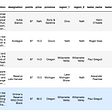## Python Pandas Tutorial (Part6)## February 26, 2018## P4 Process Book (Personal data dashboard)Hunger for Knowledge, adventure, and risk enthusiastic. Software engineering student

## Spearman Correlation in R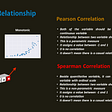## Manipulating data in R: a brief benchmark report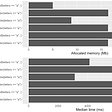## The Uses and Differences between ggplot2 and Plotly for Data Visualization with R## R package updates and release dates statistics and another rise of R language